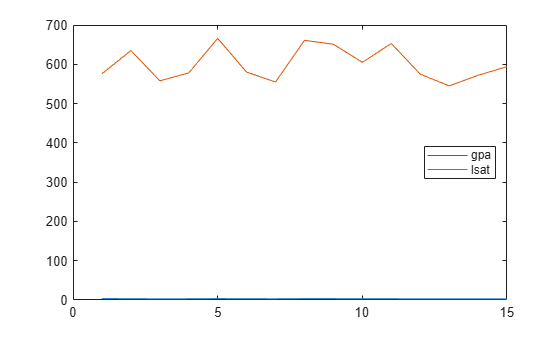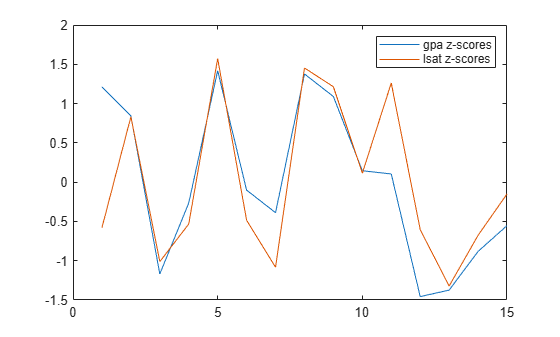Documentation

zscore

Standardized z-scores

Description

example

Z = zscore(X) returns the z-score for each element of X such that columns of X are centered to have mean 0 and scaled to have standard deviation 1. Z is the same size as X.

• If X is a vector, then Z is a vector of z-scores.

• If X is a matrix, then Z is a matrix of the same size as X, and each column of Z has mean 0 and standard deviation 1.

• For multidimensional arrays, z-scores in Z are computed along the first nonsingleton dimension of X.

example

Z = zscore(X,flag) scales X using the standard deviation indicated by flag.

• If flag is 0 (default), then zscore scales X using the sample standard deviation, with n - 1 in the denominator of the standard deviation formula. zscore(X,0) is the same as zscore(X).

• If flag is 1, then zscore scales X using the population standard deviation, with n in the denominator of standard deviation formula.

example

Z = zscore(X,flag,'all') standardizes X by using the mean and standard deviation of all the values in X.

example

Z = zscore(X,flag,dim) standardizes X along the operating dimension dim. For example, for a matrix X, if dim = 1, then zscore uses the means and standard deviations along the columns of X, if dim = 2, then zscore uses the means and standard deviations along the rows of X.

example

Z = zscore(X,flag,vecdim) standardizes X over the dimensions specified by the vector vecdim. For example, if X is a matrix, then zscore(X,0,[1 2]) is equivalent to zscore(X,0,'all') because every element of a matrix is contained in the array slice defined by dimensions 1 and 2.

example

[Z,mu,sigma] = zscore(___) also returns the means and standard deviations used for centering and scaling, mu and sigma, respectively. You can use any of the input arguments in the previous syntaxes.

Examples

collapse all

Compute and plot the $z$-scores of two data vectors, and then compare the results.

Load the sample data.

Two variables load into the workspace: gpa and lsat.

Plot both variables on the same axes.

plot([gpa,lsat])
legend('gpa','lsat','Location','East')It is difficult to compare these two measures because they are on a very different scale.

Plot the $z$-scores of gpa and lsat on the same axes.

Zgpa = zscore(gpa);
Zlsat = zscore(lsat);
plot([Zgpa, Zlsat])
legend('gpa z-scores','lsat z-scores','Location','Northeast')Now, you can see the relative performance of individuals with respect to both their gpa and lsat results. For example, the third individual’s gpa and lsat results are both one standard deviation below the sample mean. The eleventh individual’s gpa is around the sample mean but has an lsat score almost 1.25 standard deviations above the sample average.

Check the mean and standard deviation of the $z$-scores you created.

mean([Zgpa,Zlsat])
ans = 1×2
10-14 ×

-0.1088    0.0357

std([Zgpa,Zlsat])
ans = 1×2

1     1

By definition, $z$-scores of gpa and lsat have mean 0 and standard deviation 1.

Load the sample data.

Two variables load into the workspace: gpa and lsat.

Compute the $z$-scores of gpa using the population formula for standard deviation.

Z1 = zscore(gpa,1); % population formula
Z0 = zscore(gpa,0); % sample formula
disp([Z1 Z0])
1.2554    1.2128
0.8728    0.8432
-1.2100   -1.1690
-0.2749   -0.2656
1.4679    1.4181
-0.1049   -0.1013
-0.4024   -0.3888
1.4254    1.3771
1.1279    1.0896
0.1502    0.1451
0.1077    0.1040
-1.5076   -1.4565
-1.4226   -1.3743
-0.9125   -0.8815
-0.5724   -0.5530

For a sample from a population, the population standard deviation formula with $n$ in the denominator corresponds to the maximum likelihood estimate of the population standard deviation, and might be biased. The sample standard deviation formula, on the other hand, is the unbiased estimator of the population standard deviation for a sample.

Compute $z$-scores using the mean and standard deviation computed along the columns or rows of a data matrix.

Load the sample data.

The dataset array flu is loaded in the workplace. flu has 52 observations on 11 variables. The first variable contains dates (in weeks). The other variables contain the flu estimates for different regions in the U.S.

Convert the dataset array to a data matrix.

flu2 = double(flu(:,2:end));

The new data matrix, flu2, is a 52-by-10 double data matrix. The rows correspond to the weeks and the columns correspond to the U.S. regions in the data set array flu.

Standardize the flu estimate for each region (the columns of flu2).

Z1 = zscore(flu2,[ ],1);

You can see the $z$-scores in the variable editor by double-clicking on the matrix Z1 created in the workspace.

Standardize the flu estimate for each week (the rows of flu2).

Z2 = zscore(flu2,[ ],2);

Find the z-scores of a multidimensional array by specifying to standardize the data along different dimensions. Compare the results when using the 'all', dim, and vecdim input arguments.

Create a 3-by-4-by-2 array.

X = reshape(1:24,[3 4 2])
X =
X(:,:,1) =

1     4     7    10
2     5     8    11
3     6     9    12

X(:,:,2) =

13    16    19    22
14    17    20    23
15    18    21    24

Standardize X by using the mean and standard deviation of all the values in X.

Zall = zscore(X,0,'all')
Zall =
Zall(:,:,1) =

-1.6263   -1.2021   -0.7778   -0.3536
-1.4849   -1.0607   -0.6364   -0.2121
-1.3435   -0.9192   -0.4950   -0.0707

Zall(:,:,2) =

0.0707    0.4950    0.9192    1.3435
0.2121    0.6364    1.0607    1.4849
0.3536    0.7778    1.2021    1.6263

The resulting multidimensional array of z-scores has mean 0 and standard deviation 1. For example, compute the mean and standard deviation of Zall.

mZall = mean(Zall(:,:,:),'all')
mZall = -9.2519e-18
sZall = std(Zall(:,:,:),0,'all')
sZall = 1.0000

Now standardize X along the second dimension.

Zdim = zscore(X,0,2)
Zdim =
Zdim(:,:,1) =

-1.1619   -0.3873    0.3873    1.1619
-1.1619   -0.3873    0.3873    1.1619
-1.1619   -0.3873    0.3873    1.1619

Zdim(:,:,2) =

-1.1619   -0.3873    0.3873    1.1619
-1.1619   -0.3873    0.3873    1.1619
-1.1619   -0.3873    0.3873    1.1619

The elements in each row of each page of Zdim have mean 0 and standard deviation 1. For example, compute the mean and standard deviation of the first row of the second page of Zdim.

mZdim = mean(Zdim(1,:,2),'all')
mZdim = 0
sZdim = std(Zdim(1,:,2),0,'all')
sZdim = 1

Finally, standardize X based on the second and third dimensions.

Zvecdim = zscore(X,0,[2 3])
Zvecdim =
Zvecdim(:,:,1) =

-1.4289   -1.0206   -0.6124   -0.2041
-1.4289   -1.0206   -0.6124   -0.2041
-1.4289   -1.0206   -0.6124   -0.2041

Zvecdim(:,:,2) =

0.2041    0.6124    1.0206    1.4289
0.2041    0.6124    1.0206    1.4289
0.2041    0.6124    1.0206    1.4289

The elements in each Zvecdim(i,:,:) slice have mean 0 and standard deviation 1. For example, compute the mean and standard deviation of the elements in Zvecdim(1,:,:).

mZvecdim = mean(Zvecdim(1,:,:),'all')
mZvecdim = 2.7756e-17
sZvecdim = std(Zvecdim(1,:,:),0,'all')
sZvecdim = 1

Return the mean and standard deviation used to compute the $z$-scores.

Load the sample data.

Two variables load into the workspace: gpa and lsat.

Return the $z$-scores, mean, and standard deviation of gpa.

[Z,gpamean,gpastdev] = zscore(gpa)
Z = 15×1

1.2128
0.8432
-1.1690
-0.2656
1.4181
-0.1013
-0.3888
1.3771
1.0896
0.1451
⋮

gpamean = 3.0947
gpastdev = 0.2435

Input Arguments

collapse all

Input data, specified as a vector, matrix, or multidimensional array.

Data Types: double | single

Indicator for the standard deviation used to compute the z-scores, specified as 0 or 1.

Dimension along which to calculate the z-scores of X, specified as a positive integer scalar. If you do not specify a value, then the default value is the first array dimension whose size does not equal 1.

For example, for a matrix X, if dim = 1, then zscore uses the means and standard deviations along the columns of X, and if dim = 2, then zscore uses the means and standard deviations along the rows of X.

Vector of dimensions along which to calculate the z-scores of X, specified as a positive integer vector. Each element of vecdim represents a dimension of the input array X. The output Z has the same dimensions as X, but the mean mu and standard deviation sigma each have length 1 in the operating dimensions. The other dimension lengths are the same for X, mu, and sigma.

For example, if X is a 2-by-3-by-3 array, then zscore(X,0,[1 2]) uses the means and standard deviations along the pages of X to standardize the values of X.

Data Types: single | double

Output Arguments

collapse all

z-scores, returned as a vector, matrix, or multidimensional array. Z has the same dimensions as X.

The values of Z depend on whether you specify 'all', dim, or vecdim. If you do not specify any of these input arguments, then the following conditions apply:

• If X is a vector, then Z is a vector of z-scores with mean 0 and variance 1.

• If X is an array, then zscore standardizes along the first nonsingleton dimension of X.

For an example that demonstrates the differences in Z when you use 'all', dim, and vecdim, see Z-Scores of Multidimensional Array.

Mean of X used to compute the z-scores, returned as a scalar, vector, matrix, or multidimensional array. mu has length 1 in the specified operating dimensions. The other dimension lengths are the same for X and mu.

For example, if X is a 2-by-3-by-3 array and vecdim is [1 2], then mu is a 1-by-1-by-3 array of means. Each value in mu corresponds to the mean of a page in X.Standard deviation of X used to compute the z-scores, returned as a scalar, vector, matrix, or multidimensional array. sigma has length 1 in the specified operating dimensions. The other dimension lengths are the same for X and sigma.

For example, if X is a 2-by-3-by-3 array and vecdim is [1 2], then sigma is a 1-by-1-by-3 array of standard deviations. Each value in sigma corresponds to the standard deviation of a page in X.collapse all

Z-Score

For a random variable X with mean μ and standard deviation σ, the z-score of a value x is

$z=\frac{\left(x-\mu \right)}{\sigma }.$

For sample data with mean $\overline{X}$ and standard deviation S, the z-score of a data point x is

$z=\frac{\left(x-\overline{X}\right)}{S}.$

z-scores measure the distance of a data point from the mean in terms of the standard deviation. This is also called standardization of data. The standardized data set has mean 0 and standard deviation 1, and retains the shape properties of the original data set (same skewness and kurtosis).

You can use z-scores to put data on the same scale before further analysis. This lets you to compare two or more data sets with different units.

Multidimensional Array

A multidimensional array is an array with more than two dimensions. For example, if X is a 1-by-3-by-4 array, then X is a three-dimensional array.

First Nonsingleton Dimension

A first nonsingleton dimension is the first dimension of an array whose size is not equal to 1. For example, if X is a 1-by-2-by-3-by-4 array, then the second dimension is the first nonsingleton dimension of X.

Sample Standard Deviation

The sample standard deviation S is given by

$S=\sqrt{\frac{{\sum }_{i=1}^{n}{\left({x}_{i}-\overline{X}\right)}^{2}}{n-1}}.$

S is the square root of an unbiased estimator of the variance of the population from which X is drawn, as long as X consists of independent, identically distributed samples. $\overline{X}$ is the sample mean.

Notice that the denominator in this variance formula is n – 1.

Population Standard Deviation

If the data is the entire population of values, then you can use the population standard deviation,

$\sigma =\sqrt{\frac{{\sum }_{i=1}^{n}{\left({x}_{i}-\mu \right)}^{2}}{n}}.$

If X is a random sample from a population, then the mean μ is estimated by the sample mean, and σ is the biased maximum likelihood estimator of the population standard deviation.

Notice that the denominator in this variance formula is n.

Algorithms

zscore returns NaNs for any sample containing NaNs.

zscore returns 0s for any sample that is constant (all values are the same). For example, if X is a vector of the same numeric value, then Z is a vector of 0s.